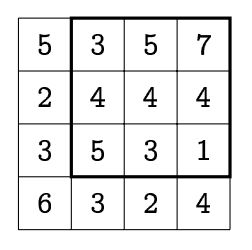시간 제한메모리 제한제출정답맞힌 사람정답 비율
3 초 128 MB192222.222%

## 문제

We are given a grid consisting of n ×m unit squares. Each of the unit squares contains a single integer. In this task we are interested in arithmetic rectangles lying on the grid, i.e., rectangles composed of unit squares such that numbers in every row and every column form arithmetic sequences. Recall that an arithmetic sequence is a sequence in which any two consecutive terms differ by the same amount.

In addition, we aim to find the largest arithmetic rectangle, i.e., the one covering the most unit squares. For example, the largest arithmetic rectangle on the grid below consists of nine unit squares:## 입력

In the first line of the input there is a single integer t (1 ≤ t ≤ 10 000) denoting the number of test cases that follow. The description of each test case begins with a line with two integers n and m (1 ≤ n, m ≤ 3 000). In each of the following n lines there are m integers from the range [0, 109]. These numbers describe the grid. The size of each input file will not exceed 20 MB.

## 출력

Your program should output t lines with answers for the consecutive test cases. The answer for a single test case is one integer equal to the number of unit squares contained in the largest arithmetic rectangle that can be found on the grid described in the test case.

## 예제 입력 1

2
4 4
5 3 5 7
2 4 4 4
3 5 3 1
6 3 2 4
2 3
0 1 2
1 2 3


## 예제 출력 1

9
6